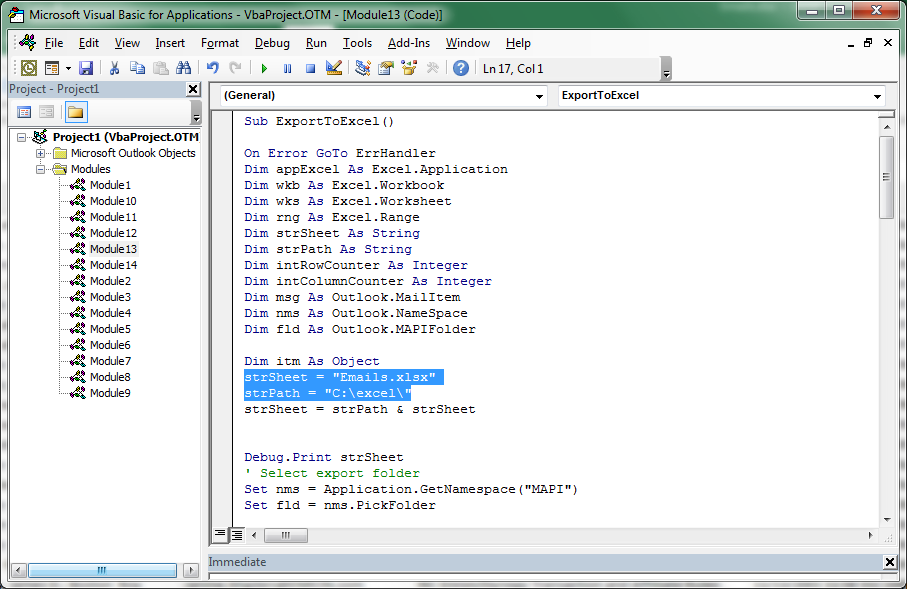top of pageSearch

# VBA code to extract metadata from Outlook emails to Excel spreadsheet

The below VBA code is based on the code posted here by Troy Graham. You can use this code to select a folder in Outlook and export the sender; to; subject; senton; and conversationindex fields to an Excel spreadsheet.

Enter Visual Basic in Outlook and paste the code in a new module. Confirm that the Microsoft Excel object library is selected in References under the Tool menu.The code requires that an Excel file be created at C:\excel named 'Emails.xlsx'. You can edit the code to alter the location of the file to which the metadata is exported.When the code is run, you will be prompted to select a folder in your Outlook profile.An Excel will be generated that will open automatically. It will have the Outlook metadata listed in separate columns.You can change the Outlook fields referenced on lines such as:

rng.value = msg.ConversationIndex

. . . . to export other metadata.

Sub ExportToExcel()

On Error GoTo ErrHandler Dim appExcel As Excel.Application Dim wkb As Excel.Workbook Dim wks As Excel.Worksheet Dim rng As Excel.Range Dim strSheet As String Dim strPath As String Dim intRowCounter As Integer Dim intColumnCounter As Integer Dim msg As Outlook.MailItem Dim nms As Outlook.NameSpace Dim fld As Outlook.MAPIFolder

Dim itm As Object strSheet = "Emails.xlsx" strPath = "C:\excel\" strSheet = strPath & strSheet

Debug.Print strSheet ' Select export folder Set nms = Application.GetNamespace("MAPI") Set fld = nms.PickFolder

' Handle potential errors with Select Folder dialog box. If fld Is Nothing Then MsgBox "There are no mail messages to export", vbOKOnly, _ "Error"

Exit Sub ElseIf fld.DefaultItemType <> olMailItem Then MsgBox "There are no mail messages to export", vbOKOnly, _ "Error"

Exit Sub

ElseIf fld.Items.Count = 0 Then MsgBox "There are no mail messages to export", vbOKOnly, _ "Error" Exit Sub

End If ' Open and activate Excel workbook. Set appExcel = CreateObject("Excel.Application") appExcel.Workbooks.Open (strSheet)

Set wkb = appExcel.ActiveWorkbook Set wks = wkb.Sheets(1) wks.Activate appExcel.Application.Visible = True

' Copy field items in mail folder. For Each itm In fld.Items intColumnCounter = 1 Set msg = itm intRowCounter = intRowCounter + 1 Set rng = wks.Cells(intRowCounter, intColumnCounter) rng.value = msg.To intColumnCounter = intColumnCounter + 1

Set rng = wks.Cells(intRowCounter, intColumnCounter) rng.value = msg.SenderEmailAddress intColumnCounter = intColumnCounter + 1

Set rng = wks.Cells(intRowCounter, intColumnCounter)

rng.value = msg.Subject intColumnCounter = intColumnCounter + 1 Set rng = wks.Cells(intRowCounter, intColumnCounter) rng.value = msg.SentOn intColumnCounter = intColumnCounter + 1 Set rng = wks.Cells(intRowCounter, intColumnCounter) rng.value = msg.ConversationIndex

Next itm Set appExcel = Nothing Set wkb = Nothing Set wks = Nothing Set rng = Nothing Set msg = Nothing Set nms = Nothing Set fld = Nothing Set itm = Nothing

Exit Sub

ErrHandler: If Err.Number = 1004 Then MsgBox strSheet & " doesn't exist", vbOKOnly, _ "Error"

Else

MsgBox Err.Number & "; Description: ", vbOKOnly, _ "Error"

End If

Set appExcel = Nothing Set wkb = Nothing Set wks = Nothing Set rng = Nothing Set msg = Nothing Set nms = Nothing Set fld = Nothing Set itm = Nothing End Sub

bottom of page